# VariableResistor

Ideal linear electrical resistor with variable resistance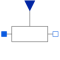# Information

This information is part of the Modelica Standard Library maintained by the Modelica Association.

The linear resistor connects the branch voltage v with the branch current i by
i*R = v
The Resistance R is given as input signal.

Attention!!!
It is recommended that the R signal should not cross the zero value. Otherwise depending on the surrounding circuit the probability of singularities is high.

# Parameters (4)

useHeatPort Value: false Type: Boolean Description: =true, if heatPort is enabled Value: T_ref Type: Temperature (K) Description: Fixed device temperature if useHeatPort = false Value: 300.15 Type: Temperature (K) Description: Reference temperature Value: 0 Type: LinearTemperatureCoefficient (¹/K) Description: Temperature coefficient of resistance (R_actual = R*(1 + alpha*(T_heatPort - T_ref))

# Connectors (4)

p n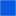Type: PositivePin Description: Positive electrical pin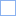Type: NegativePin Description: Negative electrical pin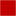Type: HeatPort_a Description: Conditional heat port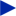Type: RealInput

# Used in Examples (5)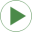ShowVariableResistor Modelica.Electrical.Analog.Examples Simple demo of a VariableResistor modelSMPM_Braking Modelica.Electrical.Machines.Examples.SynchronousInductionMachines Test example: PermanentMagnetSynchronousInductionMachine acting as brakeDCSE_Start Modelica.Electrical.Machines.Examples.DCMachines Test example: DC with serial excitation starting with voltage rampDCSE_SinglePhase Modelica.Electrical.Machines.Examples.DCMachines Test example: DC with serial excitation starting with voltage rampSMPM_Braking Modelica.Magnetic.FundamentalWave.Examples.BasicMachines Test example: PermanentMagnetSynchronousInductionMachine acting as brake

# Used in Components (1)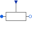VariableResistor Modelica.Electrical.MultiPhase.Basic Ideal linear electrical resistors with variable resistance